Reach Us+441474556909
Aeronautical Lithium Cobalt oxide Battery Capacity Check and Safety Anticipation Method Study | OMICS International
Journal of Electrical & Electronic Systems
All submissions of the EM system will be redirected to Online Manuscript Submission System. Authors are requested to submit articles directly to Online Manuscript Submission System of respective journal.

# Aeronautical Lithium Cobalt oxide Battery Capacity Check and Safety Anticipation Method Study

Wang SL1*Shang LP1, Fernandez C2, Li ZF3,  a Li JC4

1School of Information Engineering and Robot Technology Used for Special Environment Key Laboratory of Sichuan Province, Southwest University of Science and Technology, Mianyang 621010, China

2School of Pharmacy and Life Sciences, Robert Gordon University, Aberdeen AB10-7GJ, UK

3School of Manufacturing Science and Engineering, Southwest University of Science and Technology, Mianyang 621010, China

4MianYang Product Quality Supervision and Inspection Institute, Mianyang 621000, China

*Corresponding Author:
Wang SL
School of Information Engineering & Robot Technology
Used for Special Environment Key Laboratory of Sichuan Province
Southwest University of Science and Technology
Mianyang 621010, China
Tel: +86-15884655563
E-mail: [email protected]

Received date June 23, 2016; Accepted date July 18, 2016; Published date July 30, 2016

Citation: Wang SL, Shang LP, Fernandez C, Li ZF, Li JC (2016) Aeronautical Lithium Cobalt oxide Battery Capacity Check and Safety Anticipation Method Study. J Electr Electron Syst 5:190. doi: 10.4172/2332-0796.1000190

Copyright: © 2016 Wang SL, et al. This is an open-access article distributed under the terms of the Creative Commons Attribution License, which permits unrestricted use, distribution, and reproduction in any medium, provided the original author and source are credited.

Visit for more related articles at Journal of Electrical & Electronic Systems

#### Abstract

A novel complex equivalent circuit model has been proposed for the lithium-ion batteries. The lithium-ion battery pack capacity check method is proposed, which is based on the balance charge and serial-discharge mechanism, aiming to realize the aeronautical battery pack capacity fading and safety judgment. The method achieves the battery pack capacity check and safety anticipation maintenance by recycling balance charge and serial discharge process together with the minimum mean square error method. Experimental results show that, the method can effectively realize the capacity check and security anticipation and the accurate rate is over than 95%. The proposed capacity inspection and safety anticipation method can effectively solve the aeronautical lithium-ion battery pack capacity security prediction problem, effectively ensuring its cruising ability and security in the aero power supply applications.

#### Keywords

Aeronautical lithium-ion battery; Capacity state; Safety anticipation; Minimum mean square error; Improved equivalent circuit model

#### Introduction

The capacity state anticipation is of great importance in the LIB power application process, which is a core aspect for the modern BMS, determining whether the LIB is appropriate to continue to use or should be scrapped. It is related to the aero security, resource efficiency and environment protection issues in the power supply process. Dynamic estimation models have been studied for the capacity state prediction, but the estimation accuracy suffers from the detection errors that are not negligible. The aeronautical LIB capacity check and safety anticipation method is studied in this paper and the symbols used here can be described in Table 1.

Related researches have been done in the LIB application course, such as SOC, SOH, SOL, SOF and other aspects with in-depth studies, which effectively promote the development of the LIB industry. However, the research on the capacity check, secure prediction and their industrial application yet has no clear reports. The capacity estimation is of great significance for the safety operation of LIB packs. LIBs have many advantages compared with traditional lead-acid and nickel-cadmium batteries, such as high-energy density, long cycle life, high output voltage, low self-discharge rate, and high reliability among others. They are widely used in EVs, consumer electronics, communications, navigation and aviation among others. Especially, the LIB packs have become the third generation of aerospace and satellite power storage batteries, because they can improve the load efficiency significantly and reduce the total weight effectively.

Symbol Description Symbol Description
LIB Lithium-ion battery EKF Extended Kalman filtering
KF Kalman filtering MSE Mean square error
SOC State of charge BMS Battery management system
SVM Support vector machine RMSE Root mean square error
OCV Open circuit voltage EMF Electro-motive force
SOH State of health Ah Ampere hour
ECM Equivalent circuit model MMSE Minimum mean square error
SOL State of life SOF State of function
EVs Electric vehicles UKF Unscented Kalman filter
SOP State of power SOE State of energy
NN Neural network ANN Artificial neural network
SOB State of balance BMTS Battery maintenance and testing system
UKF Unscented Kalman filter LIBs Lithium-ion batteries
CC Constant current CV Constant voltage

Table 1:List of symbols.

The SOC is used for the capacity characterization, which attracts great attention for the LIB applications. The SOC estimation  of LIB is studied by using the square root spherical UKF method in the nanosatellite power supplies. Experimental study has been conducted on the influence of temperature and SOC on the thermophysical properties of an LIB pouch cell . The LIB thermal and electrochemical model-based state estimation  can be realized by using orthogonal collocation and a modified EKF. The ECM model with variable effective capacity is proposed by Cecilio et al.  for LiFePO4 batteries. The improved adaptive SOC estimation is proposed by Fang et al.  for batteries using a multi-model approach. The critical review of onboard capacity estimation techniques are expanded  for LIBs in electric and hybrid EVs. The SOC value is estimated by Gallien et al.  for the Magnetism versus LiFePO4 battery which is a feasibility study for magnetic-based charge monitoring. The SOC estimation is studied by He et al.  for LIBs using NN modeling and UKF-based error cancellation. A Rayleigh quotient-based recursive total-leastsquares online maximum capacity estimation is proposed by Kim et al.  for LIBs. The discrete wavelet transform-based denoising technique is studied by Lee et al.  for advanced SOC estimator of a LIB in EVs. The SOC estimation of LIB cell was reported by Tanim et al.  based on a temperature dependent and electrolyte enhanced single particle model. The Battery SOC estimation was achieved by Unterrieder et al. , using approximate least squares. The SOC estimation was stated by Xu et al.  for LIBs, which was based on a proportional-integral observer. The robust and the SOC were estimated adaptively by Zhang et al. .

Aiming to make the power supply process to be of safe and reliable, the LIB working characteristics were optimised by repeating the experiments a high number of times. A study on the impact of LIB cell relaxation on electrochemical impedance spectroscopy has been conducted by Barai et al. . The performance comparison of four LIB technologies under calendar aging was investigated by Eddahech et al. . Adaptive approach was proposed by Farmann et al.  for onboard impedance parameters and voltage estimation of LIBs in EVs. Online identification of LIB parameters were conducted by Feng et al.  which was based on an improved ECM and its implementation on the battery SOP prediction. Thermal runaway propagation model was carried out by Feng et al.  for designing a safer battery pack with 25 Ah LiNixCoyMnzO2 large format LIBs. Robust recursive impedance estimation was achieved by Fridholm et al.  for automotive LIBs. A novel Gaussian model based battery state estimation approach was constructed by He et al.  and was named as SOE. Capacity fade estimation was reported by Hussein et al.  in LIBs of EV by using ANNs. The investigation characteristics on internal short circuits of LIBs were investigated by Kim et al.  With a ceramic-coated separator during nail penetration. The aging formula was studied by Tanim et al.  for LIBs with solid electrolyte interphase layer growth.

The health state of LIB is of great importance to its power supply use, as a result, a wide variety of research have been conducted on the SOH prediction of LIBs. The SOH estimation in composite electrode with LIB cells has been conducted by Bartlett et al. . Discrimination of the degradation processes in LIB cells have been conducted by Bauer et al. , which are based on the sensitivity of aging indicators towards capacity loss. The estimation of SOC, unknown nonlinearities, and SOH for LIB was reported by Gholizadeh et al. , which is based on a comprehensive unobservable model. A multiscale Gaussian process regression modeling approach was stated by He et al.  which is used for the SOH estimation of LIBs. The inline SOH estimation was carried out by Sepasi et al.  for LIBs by using the SOC calculation. A multi time-scale SOC and SOH estimation framework was constructed by Yin et al.  by using the nonlinear predictive filter for the LIB pack with passive balance control. SOH monitoring and prediction of LIB was described by Yu et al.  by using the probabilistic indication and the state-space model.

The working state adjustment is necessary for the BMS, in which the control strategies can be designed and implemented. A novel LIB balancing strategy was proposed by Shang et al.  based on global best-first and integrated imbalance calculation. A statistical modelbased cell-to-cell variability management was constructed by Shin et al.  for the LIB pack. An adaptive nonlinear model-based fault diagnosis is proposed by Sidhu et al.  of LIBs. The online dynamic equalization adjustment was carried out by Wang et al.  of highpower LIB packs based on the SOB estimation.

The efficiency and accuracy of the algorithm are two important indicators of its performance, which are also antithetical with each other. The optimal estimation algorithm represents an obvious progress in terms of the LIB working state estimation. Since the LIBs show obvious nonlinear dynamic characteristics, the linear estimation method should be extended for suiting the nonlinear working state estimation. Some important parameters in the ECM show some timevarying characteristics because of the dynamic working conditions, such as polarization resistance and capacitance among others. The EKF and UKF that are based on the KF algorithm can better solve the nonlinear LIB working state estimation problems, which can also obtain very good estimation results.

To the best of our knowledge, we present for the first time a method to protect the safety of the LIB pack in the power supply applications, the capacity check and security prediction method. Furthermore, the LIB charge and discharge recycling maintenance were evaluated as well as predict its capacity. We also demonstrated that the method proposed in this manuscript improved the LIB life and the capacity utilization efficiency.

#### Theoretical Analysis

The filter or multiple-filter integrated algorithms based on the model construction attracts great attention, compared with the present integral method or voltage detection method. The reason is that these approaches can avoid the problems effectively from the detection noise, in-accurate sensor inputs and accumulated error among others. When doing the working state estimation by the traditional Ah integral methods, the estimation error increases conspicuously due to the accumulated error that is introduced by the current or voltage measurement noise. In order to solve these problems, the estimation model based on MMSE is constructed to improve the accuracy of the capacity estimation against the parameter perturbations caused by the measurement noise. Regardless of the aircraft topology, the primary objective of the BMS control strategy is used, aiming to satisfy the power demand of the various equipments in the aircraft control system. To respect the physical limitations imposed by the transmission components, the state variables are subject to constraint. In particular, the battery available power must be limited, in order to prevent the abuse and aging-related issues. By doing the capacity check and the safety anticipation process, the question of: “how long the battery is going to last?” It can be further predicted.

Lithium cobalt oxide principle

The LIB provides power to the outside through its discharge process, in which the two electrodes are connected through the load to form a closed circuit. Because of the potential difference effect between these two electrodes outside the battery cell, the electrons travel from the anode toward the cathode. At the same time, the lithium ions travel from the negative electrode via the electrolyte and the separator to the positive electrode in the internal LIBs, as depicted in Figure 1.

The effect of litigation on micro-scale fracture toughness of LIB cathode was reported by Mughal et al. , in which the working state characteristics under different SOC conditions are obtained and analyzed. The evaluation of electrode materials for all-copper hybrid flow batteries, in which the ion movement rule is described in Figure 1 of the referenced paper, was conducted by Leung et al. . The lithium ions will not stop in the charge or discharge process until the lithium lean or saturation state happens in the positive or the negative electrode. Equation 1 illustrates the chemical reaction described in the process.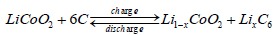(1)

Aiming to satisfy the power supply application target, the LIBs store and offer electricity through the charge and discharge processes, the transformation of which is conducted between the chemical and the electrical energy. The performance difference among the LIB cells cannot be eliminated due to the cell material and the varying production process. As a result, the LIB pack imbalance exists among the cells when they are connected together for the high-voltage power supply purpose. The recovery strategy and mechanism of aged LIBs are studied by Cui et al.  after the shallow depth of discharge at elevated temperature. In the charge and discharge process, and especially after long-term use, this imbalance will be more obvious gradually.

The experimental study is conducted for the key performance indicators of LIB packs, such as single resistance, capacitance uniformity, voltage, capacity, charge retention capability, cycle life, insulation resistance, etc., which will change with different chargedischarge rate, temperature and other conditions. Based on the direct measurement method, the key performance indicators to measure the feasibility directly or indirectly, is aiming to obtain the indirect key performance evaluation model of high-power LIB packs. The influence of memory effect on the state-of-charge estimation was reported by Shi et al.  for the large format LIBs based on the LiFePO4 cathode, in which the experimental results show that the memory effect should be taken into consideration in the battery ECM construction and capacity state estimation.

The targeted ECM is designed and introduced to simulate the effect of key reactions within the battery cell, which is based on the characteristics of the battery cell analysis results. The key characterization methods of polarization, combined with the advantageous analysis of Thevenin, PNGV, RC and a variety of improved extended ECM, by using a combination of circuit components, and the condition characteristics. The comparisons of modeling and SOC estimation was conducted by Xiao et al.  for LIB based on fractional order and integral order methods, in which the Thevenin ECM model is used and the state-space function is obtained and analyzed. The situational and experimental analysis was carried out to evaluate the equivalent simulation effect, exploring the polarized effect in the inner of the battery and self-discharge difference by using the modular modeling characterization methods. The circuit simulation was conducted to consider the internal principle and working condition analysis of the aviation LIB object, which was optimized by the combination of the equivalent description and the improvement of ECM design for the LIB pack. The internal reaction and output characteristics of the LIBs are simulated by the equivalent simulation method, which is shown in Figure 2.

In Figure 2 illustrates the improved method for ECM in the LIBs system. The parameter RS is used as the large resistance, which is designed for the self-discharge effect characterization. The parameter C1 is used as the large capacitance, which is designed for the surface effect characterization. RΩ is used as the ohmic resistance, which is intended for the inherent resistance characterization. RP is defined as the polarization resistance, which is used for the nonlinear contact resistance characterization between the electrolyte and the electrode plate. CP is the polarized condenser, which is designed to characterize the equivalent capacitance between the positive and negative plates. Rd is defined as the discharge resistance, which is designed for the increased internal resistance characterization compared with the original resistance when doing the discharging maintenance process. Rc is the discharge resistance, which is designed for the increased internal resistance characterization compared with the original resistance when doing the charge maintenance process.

By comparing the proposed ECM method with the Randle model used by Nejad et al.  the self-discharge, charge-discharge difference and the polarization effect characteristics were characterized by the proposed ECM, which is also simplified comparing with the n-RC model. The state-space description for the working process of LIBs is shown in Eq.2, in which the capacity state estimation is affected by the parameter distribution.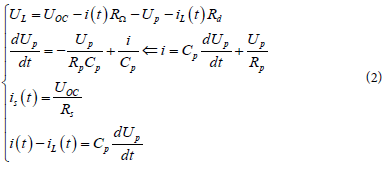There are some advantages compared with the ECM used by Lin et al.  in which a novel multi-model probability battery SOC estimation approach is proposed for EVs by using the H-infinity algorithm. The proposed ECM considered the self-discharge and charge-discharge difference characteristics of the LIB but has more simplified structure. The experimental results showed in Figure 3 are in good agreement, which shows that the SOC estimation profiles have a good effect on charactering the working principles of LIB.

MMSE nonlinear capacity estimation

The MMSE algorithm can be used in the capacity estimation and safety anticipation process of the LIB pack because of its short timeconsuming calculation characteristics, which can be realized with low demand of the hardware processors. The control strategies are studied by Torreglosa et al.  based on techno-economic optimization of renewable hybrid energy system for stand-alone applications, in which the behaviour of the energy consumption characteristics are analyzed by doing a series of experiments. The nonlinear characteristics can be described by using the MMSE method between the capacity state and the parameters of the voltage, current. Since the capacity cannot be detected directly by the measurement equipment, it can only be estimated by other parameters such as voltage, current and resistance among others which can be measured directly. Because these parameters can be considered as random variables or unknown deterministic variables, the optimal estimation should be carried out for the factor on the certain minimum performance measurement significance. By selecting a certain function of the observer parameter to make the performance value to be minimized, the target parameter value can be estimated.

Unlike other linear systems, the capacity of LIB is not only the integral of current but also the integral of voltage, internal resistance and temperature, forming the nonlinearity of the LIB packs. As there are accumulated errors caused by the current or voltage measurement noise, this joint estimator based on MMSE is proposed for the capacity estimation. The factor X should be estimated as it cannot be measured directly. Since the random parameter Z can be measured directly which is related with the target factor X, the parameter X can be realized by the estimation process of the parameter Z. The estimation value of the parameter X is a function of the parameter Z, the performance of which can be expressed as shown in the first part of Eq.2. As a result, the estimation value of the target factor is also a random variable. The function g(·) can be a nonlinear function, which can be set as a certain form function of the observer parameter Z. The estimation error can be defined as the difference value of the true value parameter X and its estimation value, which can be expressed by Eq.3.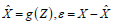(3)

The estimation error is also a random parameter which can be used to evaluate the estimation quality. Furthermore, the estimation error will be expected to be with zero means and minimal variance, which is a special circumstance of the Bayesian performance measurement. The performance measurement parameter J is the criterion used for justifying the estimation optimality, which has mounts of function manifestations. The most common form is the expectation of the cost function C(·), which is also a function of the difference between the actual value and estimated value. This Bayesian performance measurement can be expressed in Eq.4.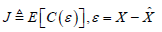(4)

In the above formula, the Bayesian estimation from the parameter Z to the parameter X can be obtained according to Eq.1, in which the function g(Z) is used to make the parameter J to be minimal. Herein, the performance measurement uses the MSE method which can be described as shown in Eq.5, in which the function g(Z) is the MSE error of the parameter X.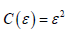(5)

When estimating the target random variables by using other random factors, this MSE criterion can play effective role in solving the question and obtain the correct mathematical solutions.

Knowing that the LIB capacity states estimation, it is assumed that the LIB power supply system is a Gaussian system and the Markov sequence model can be used for the state estimation which can be expressed in the form of a differential matrix equation as shown in Eq.6.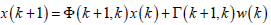(6)

Wherein, the sequence vector parameter x(k) is defined as the output of the dynamic system, using the white noise process vector w(k) as the model input. The coefficient matrix parameter φ(k+1,k) is a vector with n*n dimensions and the coefficient matrix parameter Ð³(k+1,k) is a vector with n*p dimension. The noise parameter w(k) is a p*1 dimensional white vector sequence, the covariance matrix of which can be described that is shown in Eq.7.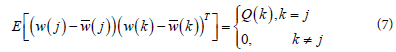Because the noise parameter w(k) is a p*1 dimensional sequence, the covariance matrix Q(k) should be a definite p*p dimensional matrix that is positive as well, the value of which is not required to be a diagonal matrix in every k time point condition. As a result, the elements in the model vector w(k) can be related. Meanwhile, as the covariance matrix Q(k) is a function of the parameter k, this model should contain the non-stationary process. The initial condition vector x(0) is set as a random vector with n dimensions. The n*1 dimensional mean vector E[x(0)] together with the n*n dimensional covariance matrix P(0) can be incited as shows in Eq.8.To simplify the calculations, the white sequence w(k) is assumed to be irrelevant with the initial condition vector x(0) at the arbitrary k time point, satisfying the condition equation that is shown in Eq.9.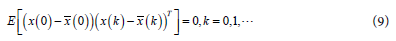In the measurement process, because there are measurement errors in the detection, the system state cannot be measured as error-free measurements, and therefore the observation vector can be obtained containing measurement errors, which is shown in Eq.10.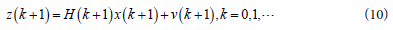Wherein, the vector z(k+1) is a m dimensional vector, which represents the observation vector at the k+1 time moment. The vector x(k+1) is a n dimensional vector, which indicates the system state vector at the k+1 time moment. The vector H(k+1) is a m*n dimensional vector, which indicates the measurement vector at the k+1 time moment. The vector v(k+1) is a m dimensional vector indicating the time measurement error. The initial value of the state vector x(0) is an initial condition with its mean matrix and the covariance of which can be described by using the matrix P(0). It is assumed that the observation error vector v(k+1) is a white random process, the mean and covariance matrix of which can be described by Eq.11.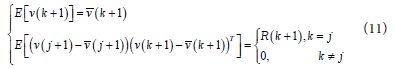Wherein, the matrix R(k) is the m×m dimensional covariance matrix without the diagonalization converting process and it is a function of time. Therefore, whether the observation error vector is a smooth process or not is without request. Meanwhile, in order to simplify the calculation, it is assumed that the vector v(k+1) is not related to the initial state condition x(0), and the vector v(k+1) is irrelevant to the vector w(j+1) for all j and k values, meeting the conditions that are shown in Eq.12.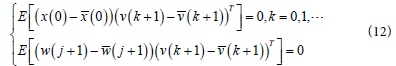In the estimation process, the observation vector z(1), z(2), …, z(j) are estimated at the given moment 1, 2, …, j conditions. The function values of these observations are estimated by the state vector x(k), which is characterized by the estimation value of the parameter x(k|j), which can be described by Eq.13.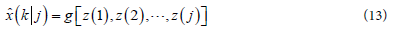When the vector x(k) is estimated by using the given j dimensional observation vector, it will generate the estimation error, the vector of which can be defined by Eq.14.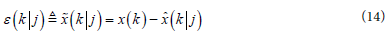Because the estimation function of the parameter x(k|j) is a random process of the vector x(k) and the vector z(j), its error is also a random process. The method used to estimate the performance results in this paper is represented by the evaluation of the error function C(*) for the expected demand, which is shown in Eq.15.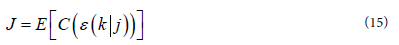The function g(·) concerning the observation values z(1), z(2), …, z(j) are studied to reach the minimum of the parameter J, which is named as the best estimation of the parameter J. The different estimation results can be obtained when selecting different handling mechanism of the function C(·) in this estimation process. As a result, the MMSE algorithm is used for the estimation, the expression function of which is shown in Eq.16.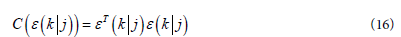The function g(·) is set as a linear function of the observation vector that is shown in Eq.5, which is expressed as the matrix product form of observations z(1), z(2), …, z(j). The observation vector z*(j) is a j·m dimensional column vector, combined and formed by the observation vector z(k), which is shown in Eq.17.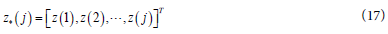The parameter z(k) is a m dimensional vector, therefore, the parameter z* (j) is a j·m dimensional column vector, which increases along with the parameter j. Furthermore, the estimated value can be described by Eq.18.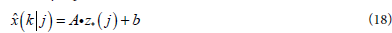In the formula, the coefficient matrix A is a n×j·m dimensional matrix and the constant vector b is a n×1 dimensional matrix. The state vector x(k) and observation error v(k) are set as zero-mean. According to the MSE criterion, the parameter C(x) in Eq.10 can be represented as C(x)=xTx, and as a result, the parameter J can be converted to Eq.19.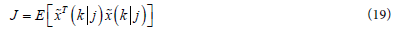According to the above enactment and calculation procedures, the constant vector b can be a zero-vector and then the problems can be converted to look for a matrix to make the parameter J to be the minimum. Since the parameter x(k) and z(k) are zero-mean random sequences, the Eq.20 can be obtained by using the orthogonal property.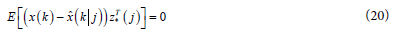In the formula above, the right zero matrix is a n×m·k dimensional matrix and all the elements are initialed with zero value. Similarly, the MMSE value can be obtained by the orthogonal guideline, which is shown in Eq.21.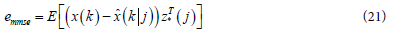By introducing Eq.18 into Eq.20, the relationship between the vector x and the vector z can be obtained, which is shown in Eq.22.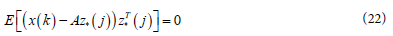The expectation can be obtained by Eq.23, which shows the expectation and the calculation analysis.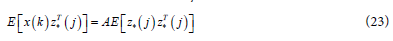In the above formula, the coefficient matrix A can be calculated as shown in Eq.24.Therefore, the best linearity estimation can be obtained by using the MMSE formula, which is described by Eq.25.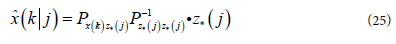Security anticipation model

The real-time security prediction of LIB is to estimate the future value based on the current and past observations in the maintenance process. The available observation can be obtained by the real-time detection that has a statistical relationship with the state vector x(k) that is shown in the parameter z(k) of Eq.5, the target of which is to get the forecast estimation parameter x(k+N|k) for the state vector x(k+N). Herein, the previous time of the prediction is a fixed integer, the fixed previous forecast process of which can be conducted. The new observation will be obtained at each sampling time point, making all the observation steps use a fixed ahead time stage in the prediction process.

The system state and observation equations are constructed for the estimation process, which are shown in Eq.1 and Eq.5. In the observation equation that is shown in Eq.1, the parameters Φ(k+1,k), Γ(k+1,k), P(0) and Q(k) are known advanced which are also deterministic. In the observation equation that is shown in Eq.5, the parameters of H(k+1) and v(k+1) are known as deterministic. Therefore, the expectation calculation process can be done by using the formula of the optimal fixed N ahead time points ahead forecast, which can be obtained by Eq.26.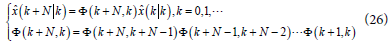Wherein, the estimation parameter of the vector x(k|k) is the optimal linear filter state estimation at k time point and the initial conditions are set as the estimation value of the vector x(0|0) and P(0|0) = P(0). In the capacity safety prediction process of LIB, the error covariance matrix is used to express the evaluation performance, the calculation process of which is shown in Eq.27.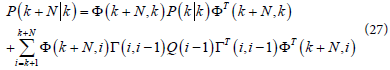Wherein, the calculation process of the summation term process and the function Φ(k+N, k) have the same recursive manner to strike. Since the MMSE based filter uses a statistical approach, the method can yield better performance and works particularly well for nonlinear and robust problems of the LIB power supply system.

#### Experiment and Analysis

Tï»¿ï»¿ï»¿ï»¿his section demonstrates how the proposed LIB capacity estimation method and the performance classification can be applied and validated. Firstly, the charging-shelve-discharging maintenance experimental research of the LIB packs is done and analyzed. Then, the capacity estimation results can be obtained which are compared with the actual capacity by doing the discharge maintenance of the LIB pack. At last, the safety anticipation results are obtained which are also analyzed for the aircraft application demands.

Experimental research of LIB cycling maintenance

The experimental samples are the LIB packs with 7 serially connected cells, the rated capacity of which is 45 Ah. The nominal voltage is 25.9 V and the working temperature range is -55°C ~ 70°C. The laboratory instrument is the self-developed BMTS. The experimental ambient temperature is 25°C ± 10°C and the relative humidity is ≤80%. The fitted curve can be obtained by doing the discharge and charge maintenance that is shown in Figure 3, which has the same form roughly as the experimental results shown in Ref.12. Nevertheless, there are obvious differences at some places, such as at the time point around 150*10 s time point. The reason is that the curve used in the reference paper is the experiment from lithium iron phosphate batteries, but the lithium cobalt oxide batteries are used in our studies, and the two kinds of batteries have some different features.

The experimental equipment was designed in-house and was named BMTS, which is shown in Figure 4. The Experimental setup for capacity test and thermal cycle is also designed by Mendoza et al.  compared with which the system structure is more succinct and suitable for the equipment users.

The full charged battery is discharged in order to obtain the raw data. The discharging approach used in this experiment is a CC-CV discharging method, which is realized by using varies current digital load. The regression algorithm is used to identify piecewise the pulse load characteristics as shown in Figure 4. The regression equation is designed and described in Eq.13. The regression parameters are characterized by using the variables such as R, C, τ et al. Then, the parameters can be obtained for the battery ECM that is shown in Figure 2. The SOC estimating of LIBs using an H-infinity observer with consideration of the hysteresis characteristic is done by Xie et al. .

The battery parameters are changing slowly along with the time ranging. Thus, the update rate of the LIB parameters also including their identification algorithm need not be too fast. Two forms are performed for the battery parameter identification in the experiments, in which the identification algorithm runs once in every 300 s. The discrete wavelet transform-based feature extraction of experimental voltage signal is also done by Kim et al.  for LIB cell consistency, in which the CC-CV charge method is also used and the charge-discharge cycling maintenance is designed and conducted for obtaining the LIB working state and characteristics. The state-space model is constructed by Zou et al.  with non-integer order derivatives for LIB, in which the fractional-order model is used to characterize the working state of the LIBs.

Accordingly, the battery parameters are updated one time every 300 s. The regression algorithm is used to identify the battery parameters. The computational amount of the estimation process is a little big, which makes it difficult to obtain the battery parameters in each maintenance cycle. Fortunately, considering the slow change rate of the LIB parameters, the real-time requirements of the parameter identification algorithm is not very high, so the battery parameters are not updated in each run but only a few times in our experiments. The online internal short circuit is detected by Feng et al. for a large format LIB, in which the relationship between the capacity and voltage is studied and obtained as shown in Figure 4 . The OCV-based SOC estimation of LIB using dual NN fusion battery model is conducted by Dang et al.  in which the Thevenin model is used for the SOC estimation and the estimation curve had similar tracking results.

In the experiment, the capacity check and anticipation prediction process can be conducted in terms of the MMSE usage. The capacity of a specified LIB experiencing performance degradation can be estimated by the similar recognition of either CC-CV charging or the CC discharging maintenance process. Aiming to satisfy the verification purpose, mounts of CC-CV charging and CC discharging maintenance cycles are done in the experiment throughout the entire degradation process to estimate the corresponding capacity. In general, the discharging capacity is always reduced with the increase of the discharging rate. Higher discharging rate means a bigger current that is presented in the circuit which may generate more heat in the LIB packs. The SOC estimation of LIB was conducted by Meng et al. , which is based on the adaptive UKF and SVM. The SOC values stated by Meng et al.  showed a good tracking effect and the output voltage characteristics have similar characteristics. The proposed BMTS had more convenience than the experiment equipment.

The actual capacity state of an arbitrary LIB can be recognized by composing a certain state of charging or discharging maintenance on it. Consequently, a unique corresponding CC-CV maintenance curve can be determined for a certain charging-discharging cycle under a specific operating condition. A systematic SOC estimation framework is proposed by Sun et al.  for the multi-cell battery pack in EVs using bias correction technique, in which the SOC difference characteristics among the connected cells are studied. The LIB pack suffers from the consistency difference among the connected cells as reported from Sue et al. when the max available capacity state is of 12 cells, The shape and the structure of the cycling process including the shelve time between the charge and charge stages can reflect the LIB actual capacity state. Thus, the similarity of the pair-wise curves derived from every two states of the LIB pack reflects the similarity of these two states. Furthermore, external interferences affect the state cognition process and the accuracy of the curve similarity computation. The variance voltage of the single one LIB cell has been enlarged in the figure comprehensively in order to understand the cell characteristics better. The model filter based on MMSE is used for the simultaneous capacity estimation to improve its accuracy .

The experimental results are analyzed by comparing with each DCM results, in which the similar varying presences are obtained in the experiments. The voltage and current characteristics of the LIB pack and cells are shown separately in Figures 4-6, where the different color lines present the varying cell numbers. It can be found that although every cell has similar charging and discharging variation, there are also some differences among the connected LIB cells, as can be seen in the enlarged areas in the figures because of the material and manufacturing process variations. The particle-filtering-based estimation was conducted by Burgos-Mellado et al.  for determining the maximum available power state in LIBs.

The capacity estimation of LIB packs

To verify the applicability of the joint estimation algorithm based on the MMSE method, the 7ICP45 LIB packs were used in the experiments, the nominal capacity of which is 45 Ah. The estimation effect was analyzed when the SOC initial value was small. The actual SOC initial value was set as 0.4 and the initial SOC filter value was set as 0.3. The prior-knowledge-independent equalization was obtained to improve the battery uniformity with energy efficiency and time efficiency for LIB by Zhang et al. , which provided the consultation of the capacity state estimation for the group work LIB pack. A mixed SOC estimation algorithm was proposed by Lim et al.  with high accuracy in various driving patterns of EVs, compared to the SOC estimation results obtained in this paper which had better astringency. Figure 7 shows the estimated effect analyzed and the absolute error curve of the SOC estimation obtained.

As can be seen from Figure 7, when the SOC initial value of the constructed filter is small and close to the actual SOC value, the convergence speed is very fast. A comparative study was conducted by Yang et al.  of three model-based algorithms for estimating SOC of LIBs under a new combined dynamic loading profile, in which the SOC estimation had similar experimental values compared to the results reported in this manuscript. In this case, the SOC estimation had high estimation accuracy, in which the maximum absolute error was only 1.83%. Although the SOC estimation curve still appears some jitter at some times, the error is very small and the impact is not great. The SOC estimation results are in good agreement with those reported by El Mejdoubi et al.  in which the LIB diagnosis according to surface temperature variation, the correctness and accuracy of the proposed ECM and estimation model can be proved. The actual initial value will be corrected in the estimation process so that the jitter is negligible. As can be known from the simulation analysis, the lower the actual initial SOC value, the smaller the estimated absolute error is, which makes the estimation result to be better .

#### Conclusion

The cycling charge, shelve and discharge maintenance has been achieved to characterize the aircraft LIB packs, which is beneficial to simplify the estimation algorithm structure. According to the perspective of the system approximation, when the MMSE method follows the safety prediction model, the linearization method keeps the effect trend essentially. This derives from the estimation model and subsequently acts on the estimating increment of the capacity state. Moreover, the improved ECM and MMSE algorithms were adopted to construct the capacity state estimation model for the LIB packs. The effectiveness of this algorithm was verified experimentally through fully and partially battery charge and discharge experiments.

#### Acknowledgements

The authors would like to express their gratitude to Sichuan Science and Technology Support Program (No. 2014GZ0078) and Mianyang Science and Technology Project (No. 15G-03-3).

Select your language of interest to view the total content in your interested language

### Article Usage

• Total views: 8996
• [From(publication date):
September-2016 - Sep 18, 2019]
• Breakdown by view type
• HTML page views : 8904

## Post your commentCan't read the image? click here to refresh
###### Peer Reviewed Journals

Make the best use of Scientific Research and information from our 700 + peer reviewed, Open Access Journals

### Clinical & Medical Journals

International Conferences 2019-20

Meet Inspiring Speakers and Experts at our 3000+ Global Annual Meetings

Top